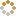﻿ Ningbo University of Technology Online Judge ::  Enumerate the Triangles
• ###  Enumerate the Triangles

• 时间限制: 2000 ms　内存限制: 32768 K
• 问题描述
• Little E is doing geometry works. After drawing a lot of points on a plane, he want to enumerate all the triangles which the vertexes are three of the points to find out the one with minimum perimeter. Your task is to implement his work.
• 输入
• The input contains several test cases. The first line of input contains only one integer denoting the number of test cases.
The first line of each test cases contains a single integer N, denoting the number of points. (3 <= N <= 1000)
Next N lines, each line contains two integer X and Y, denoting the coordinates of a point. (0 <= X, Y <= 1000)
• 输出
• For each test cases, output the minimum perimeter, if no triangles exist, output "No Solution".
• 样例输入
• ```2
3
0 0
1 1
2 2
4
0 0
0 2
2 1
1 1
```
• 样例输出
• ```Case 1: No Solution
Case 2: 4.650
```
• 提示
• `无`
• 来源
• `HyperHexagon’s Summer Day Gift`
• 操作
﻿